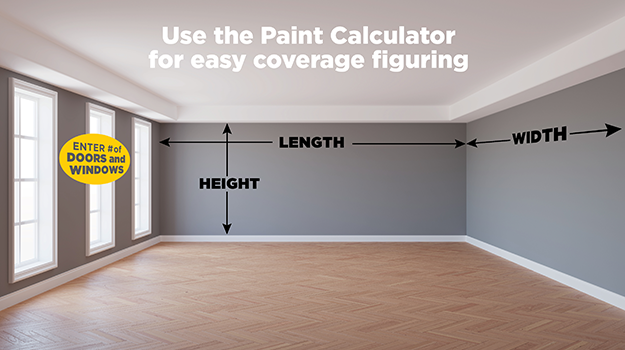Paint Calculator - Cary PaintPlus Hardware – Paint Plus Hardware - True Value Manufacturing, LLC

# Paint Calculator

## The Paint Calculator takes out the guess work behind the right amount of paint for your next project.

How Much Paint Do I Need? One gallon will cover about 350 square feet. To ﬁgure out how many gallons you will need, multiply length x height to get square footage of each wall then add together.

Or input your dimensions below into our paint calculator for a close estimate.• ft
• ft
• ft

## Exterior

• ft
• ft
* Calculation is based on the following assumptions: 1) room has 4 walls 2) a gallon of paint covers 350 square feet 3) as a rule, windows are 15 square feet and doors are 21 square feet